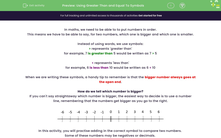# Using Greater Than and Equal To Symbols

In this worksheet, students will identify the larger number in pairs including decimals and negative numbers, using the correct symbol to define the relationship (<, >, =).Key stage:  KS 4

Year:  GCSE

GCSE Subjects:   Maths

GCSE Boards:   Pearson Edexcel, OCR, Eduqas, AQA,

Curriculum topic:   Number, Algebra

Curriculum subtopic:   Structure and Calculation Algebraic Inequalities

Popular topics:   Algebra worksheets

Difficulty level:#### Worksheet Overview

In maths, we need to be able to to put numbers in order.

This means we have to be able to say, for two numbers, which one is bigger and which one is smaller.

Instead of using words, we use symbols:

> represents 'greater than'

for example, 7 is greater than 5 would be written as 7 > 5

< represents 'less than'

for example, 6 is less than 10 would be written as 6 < 10

When we are writing these symbols, a handy tip to remember is that the bigger number always goes at the open end.

How do we tell which number is bigger?

If you can't say straightaway which number is bigger, the easiest way to decide is to use a number line, remembering that the numbers get bigger as you go to the right.In this activity, you will practise adding in the correct symbol to compare two numbers.

Some of these numbers may be negatives or decimals.

### What is EdPlace?

We're your National Curriculum aligned online education content provider helping each child succeed in English, maths and science from year 1 to GCSE. With an EdPlace account you’ll be able to track and measure progress, helping each child achieve their best. We build confidence and attainment by personalising each child’s learning at a level that suits them.

Get started